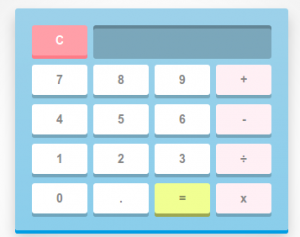# 20+ JS Calculator Scripts

JS Calculator Scripts – Are you looking for JS Calculator Script, If yes then in this post I am going to share hand-picked JS Calculator Scripts for you. Some time You have to integrate calculator to your website. As every visitor of your site wants information in figures and stats. Now, a calculator can do a lot of tasks on the front-end too. In fact, sometimes you would need this tool to present stats like BMI index, Currency calculators and more. So, these calculators will ease out your workload too! Because computing the values would not take any manual efforts. Plus, you are dead sure about getting the accurate results. You can use these JS Calculator Scripts in your next web based projects.### JS Calculator Scripts

Following are the list of JS Calculator Scripts.

#### JavaScript calculator – DEMO

Vanilla JS. Sizing is iffy on smaller devices.

#### React Calculator – DEMO

A calculator built with React

#### Simple Calculator – DEMO

Just a simple calculator, not much to describe.

#### Simple jQuery Calculator – DEMO

A simple calculator implemented using jQuery. Another FCC zipline challenge.

#### React Calculator – DEMO

Calculator is an exercise in React, playing with custom data stores and PostCSS. Click the RECALL button for a brief abstraction on a Flux store. The code for the store starts on line 177.

#### Memory-first Calculator – DEMO

Visual memory for a calculator.

#### JavaScript Calculator – DEMO

A simple calculator built using HTML, CSS and JavaScript.

#### Calculator starter – DEMO

This is the starter file for a blog post “How to build a calculator”. You can follow the lesson at https://zellwk.com/blog/calculator-part-1

#### Apple’s Calculator – DEMO

An html/css version of Apple’s Calculator. Fonts are guessed. Calculator logic done using Angular.js

#### Simple Calculator – DEMO

Simple Javascript calculator. Might have some bugs. Original js code from http://thecodeplayer.com/walkthrough/javascript-css3-calculator. Also, if anyone has a dribbble invite, https://dribbble.com/josec or [email protected]

#### calculator – DEMO

javascript calculator

#### calculator – DEMO

javascript calculator

#### JavaScript Calculator – DEMO

A simple example of building a calculator in JavaScript.

#### Bootstrap JS Calculator – DEMO

A calculator I made using Bootstrap, CSS, and JavaScript

#### Calculator – DEMO

Simple calculator using CSS and JS, free code camp project.

#### Javascript and CSS3 Calculator – DEMO

Learn to make a cool looking calculator using Javascript and CSS3.

#### Brushed Metal Calculator – DEMO

This is a Freecodecamp zipline project. Brushed metal buttons idea was taken from: http://simurai.com/lab/2011/08/21/brushed-metal/

#### JavaScript Slider Calculator – DEMO

A simple example of building a calculator with the slider using JavaScript

#### Wage Gap Calculator – DEMO

dailyui 004: Calculator is a weightier take on calculations than some pretty squares with numbers in them.

#### Javascript Calculator – DEMO

Featured on Free Code Camp @ https://www.freecodecamp.com/challenges/build-a-javascript-calculator. Built using HTML5, CSS3, JavaScript, jQuery, & Bootstrap.

#### Vue Calculator – DEMO

Simple calculator during my learning process of Vue.js 2

iOS8 inspired

#### React Calculator – DEMO

Calculator built with React.js. Inspired by http://codepen.io/tbremer/pen/wKpaWe

I Hope you liked Hand-picked list of JS Calculator Scripts, Don’t forget to Subscribe My Public Notebook for more useful Hand-picked HTML and CSS code examples, tutorials and articles.